# The Electric Field Between Two Plates: Formula, Magnitude, Direction, Imp FAQs

In this article, we will use Gauss’ law to calculate the electric field between two plates and the electric field of a capacitor.

## The electric field between two plates:

The electric field is an electric property that is linked with any charge in space. Thus, the electric field is any physical quantity that takes different values of electric force at different points in a given space.

An electric field is an area or region where every point of it experiences an electric force.

Electric fields can be described in a general way as electric force per unit charge.

If we consider an infinite plane having a uniform charge per unit area, i.e., ර, then for the infinite plane, an electric field can be given by:

Let’s look at the electric field when two charged plates are involved.

## A uniform electric field exists between two charged plates:

According to Coulomb’s law, the electric field around a point charge reduces as the distance from it rises. However, a homogeneous electric field may be created by aligning two infinitely large conducting plates parallel to each other.

“If at each point in a given space the strength of the electric field stays unchanged, then the electric field is said to be a uniform electric field.”

The field lines of a uniform electric field tend to be parallel to each other, and the space between them is also equal.

Parallel field lines and a uniform electric field between two parallel plates provide the same attraction and repulsion force on the test charge no matter where it is in the field.

Field lines are always drawn from high-potential to low-potential regions.

## The direction of an electric field between two plates:

The electric field travels from a positively charged plate to a negatively charged plate.

For example, suppose the upper plate is positive, and the lower plate is negative, then the direction of the electric field is given as shown below figure.

Positive and negative charges feel the force under the influence of the electric field, but its direction depends on the type of charge, whether positive or negative. Positive charges sense forces in the direction of the electric field, whereas negative charges feel forces in the opposite direction.

## The electric field between two parallel plates of the same charge:

Suppose we have two infinite plates which are parallel to each other, having positive charge density ර. Now, here we calculate the net electric field due to these two charged parallel plates.

Both electric fields are opposing each other in the centre of the two plates. As a result, they cancel each other out, resulting in a zero net electric field within.

∴Ein = 0

Both electric fields point in the same direction outside the plates, i.e., on the left and right sides. Thus, its vector sum will be ?/?0.

Eout = E1 + E2

## The electric field between two parallel plates of opposite charge:

Suppose we have two plates having charge densities +ර and -ර. The distance d separates these two plates.

Plate with a positive charge density produces an electric field of E=ර/2ε0. And the direction of it is in the outward direction or away from the plate, while the plate with negative charge density has an opposite direction, i.e., inward direction.

So, when we use the superposition principle at both sides of plates outside and inside the plates, then we can see that outside the plate, both electric field vectors have the same magnitude and opposite direction, and thus, both electric fields cancel each other out. So, outside of the plates, there will be no electric field.

∴Eout=0

As they support each other in the same direction, the net electric field between two plates is E=ර/ε0.

Ein = E1 + E2

This is the fact we are using to form a parallel plate capacitor.

## The electric field between two plates given voltage:

In physics, either potential difference ΔV or electric field E is used to describe any charge distribution. Potential difference ΔV is closely related to energy, while electric field E is related to the force.

E is a vector quantity, implying it has both magnitude and direction, whereas ΔV is a scalar variable with no direction.

When a voltage is applied between two conducting plates parallel to each other, it creates a uniform electric field.

The strength of the electric field is directly proportional to the applied voltage and inversely proportional to the distance between two plates.

## The electric field between two parallel plate capacitors:

### Parallel plate capacitor:

A parallel plate capacitor comprises two conducting metal plates that are connected in parallel and separated by a certain distance. A dielectric medium fills the gap between the two plates.

Dielectric medium is an insulating material, and it can be air, vacuum, or some non-conducting materials like mica, glass, electrolytic gel, paper wool, etc. Dielectric material stops current from passing through it due to its non-conducting property.

However, when voltage is applied to the parallel plates, the dielectric medium’s atoms will polarise under the effect of the electric field. The process of polarisation will form dipoles, and these positive and negative charges will accumulate on the plates of the parallel plate capacitor. A current flows through the capacitor as the charges accumulate until the potential difference between two parallel plates equalizes the source potential.

The capacitor’s electric field strength must not exceed the dielectric material’s breakdown field strength in parallel plate capacitors. If the capacitor’s operating voltage exceeds its limit, the dielectric breakdown causes a short circuit between the plates, destroying the capacitor immediately.

Thus, to protect the capacitor from such a situation, one should not exceed the applied voltage limit and choose the range of voltage capacitors.

The electric field between parallel plate capacitor:

The following figure illustrates the parallel plate capacitor.

In this case, we’ll take two large conducting plates parallel to each other and separate them by d. The gap is filled with the dielectric medium, as shown in the figure. The distance d between two plates is significantly smaller than the area of each plate. Therefore we may write d<<A.

Here, the charge density of the 1st plate is +ර, and the charge density of the 2nd plate is -ර. Plate 1 has a total charge Q, and plate 2 has a total charge -Q.

As we have seen earlier, when two parallel plates of opposite charge distribution are taken, the electric field in the outer region will be zero.

As a result, the net electric field in the center of the parallel plate capacitor may be calculated as follows:

E = E1 + E2

=ර/2ε + ර/2ε

=ර/ε

Where ර is the surface charge density of the plate

ε  is the permittivity of the dielectric material used to form capacitors.

From the above equation, we can say that the dielectric medium causes a decrease in electric field strength, but it is used to get higher capacitance and keep conducting plates coming in contact.

## The magnitude of the electric field between two charged plates :

If two indefinitely large plates are taken into consideration, no voltage is supplied, then the electric field magnitude according to the law of Gauss must be constant. But the electric field between two plates, as we stated previously, relies on the charge density of the plates.

Therefore, if two plates have the same charge densities, then the electric field between them is zero, and in the case of opposite charge densities, the electric field between two plates is given by the constant value.

When the charged plates are given a voltage, the magnitude of the electric field is decided by the potential difference between them. A higher potential difference creates a strong electric field, while a higher distance between the plates leads towards the weak electric field.

So, the distance between plates and potential difference are the essential factors for the electric field strength.

## Q. How is the electric field between parallel plates different from the electric field around a charged sphere?

Ans. The electric fields between parallel plates and around a charged sphere are not the same. Let’s see how they vary.

The electric field between parallel plates depends on the charged density of plates. If they are oppositely charged, then the field between plates is ර/ε0, and if they have some charges, then the field between them will be zero.

Outside the charged sphere, the electric field is given by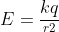whereas the field within the sphere is zero. In this case, r represents the distance between a point and the centre.

## Q. What will happen to the electric field and voltage if the distance between the plates of the capacitor is doubled?

Ans. E=ර/ε0 determines the electric field between parallel plate capacitors according to Gauss’ law.

According to Gauss’ law, the electric field remains constant since it is independent of the distance between two capacitor plates. If we talk about the potential difference, it is directly proportional to the distance between two plates of a capacitor and is given by

Thus, if the distance is doubled, then the potential difference also increases.

## Q. How do I calculate the electric field in a parallel plate capacitor?

Ans. In parallel plate capacitors, both plates are oppositely charged. Thus, the electric field outside the plates will be canceled out.

Both plates are oppositely charged, and therefore the field between plates will support each other. Moreover, between two plates dielectric medium is present, so the permittivity of dielectric will also be an essential factor.

Gauss’ law and the concept of superposition are used to calculate the electric field between two plates.

E = E1 + E2

=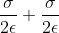=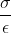Where ර is the surface charge density

ε is the permittivity of dielectric material.

## Q. Why does the electric field between the plates of capacitors decrease when introducing a dielectric slab? Explain with the help of a diagram.

Ans. When a dielectric material is placed between parallel plates of the capacitor under an external electric field, the atoms of the dielectric material will polarise.

Charge accumulation on capacitor plates is caused by induced charge in the dielectric material. As shown in the figure below, this charge accumulation causes an electric field between two plates that resist the external electric field.

The above figure shows the dielectric slab between two capacitor plates since the dielectric slab induces the opposite electric field; hence the net electric field between the capacitor plates is decreased.

## Q. Two identical metal plates are given positive charge Q1 and Q2, respectively. If they are brought together to form the parallel plate capacitor with capacitance C, the potential difference between them is ……..

Ans. The capacitance of a parallel plate capacitor, which is made up of two identical metal plates, is calculated as follows:

Where C is the parallel plate capacitor’s capacitance

A is the area of each plate

d is the distance between parallel plates

Let’s say the surface charge density is

Now, the net electric field can be given by,

Potential difference is represented by,

Thus, substituting the above values in this equation, we get a potential difference

## Q. What happens when a dielectric material is introduced between parallel plates of the capacitor?

Ans. Electric field, voltage, and capacitance change when we introduce dielectric material between parallel plates of the capacitor.

The electric field drops when a dielectric material is introduced between parallel plates of a capacitor due to charge accumulation on the parallel plates, which generates an electric field in the opposite direction of the external field.

The electric field is given by

Electric field and voltage are proportional to each other; thus, the voltage also decreases.

The capacitance of the capacitor, on the other hand, increases because it is proportional to the permittivity of the dielectric material.

## Q. Does a magnetic field exist between the plates of a capacitor?

Ans. Magnetic fields exist between two plates only when the electric field between two plates is changing.

Thus, when a capacitor is getting charged or discharged, the electric field between two plates changes, and only at that time magnetic field exists.

## Q. What happens when a high electric field is stored in a very small region of space? Is there a limit of capacitance?

Ans. Capacitors are electrical devices that use a sustained electric field to store electric charges as electrical energy. Between the capacitor’s plates lies the dielectric material.

If the applied external electric field exceeds the breakdown field strength of dielectric material, then insulating dielectric material becomes conductive. Electrical breakdown leads towards the spark between two plates, which destroys the capacitor.

Each capacitor has a different capacitance based on the dielectric material used, area of plates, and distance between them.

The tolerance of the capacitor is found anywhere between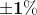to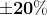of its advertised value.

## Q. What are the applications of Gauss’s law?

Ans. Gauss’ law has various applications.

In some cases, the calculation of electric fields involves tough integration, and it becomes quite complex. We use Gauss’ law to simplify the evaluation of electric fields without involving complex integration.

• The electric field at distance r in the case of an infinitely long wire is E= ?/2?ε0

Where the ? is the linear charge density of wire.

• The near-infinite planar sheet’s electric field strength is E=ර/2ε0
• The electric field strength at the spherical shell’s outer area is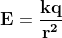and E=0 within the shell.
• The strength of electric field between two parallel plates E=ර/ε0, when the dielectric medium is there between two plates then E=ර/ε.

## Q. The formula for a parallel plate capacitance is:

Ans. By maintaining the electric field, capacitors are used to store electric charges in electrical energy.

When the plates are separated by air or space, the formula for a parallel plate capacitor is:

, Where C is the capacitor’s capacitance.

Scroll to Top